# Python 学习(一)

#### 1. 保留字

['False', 'None', 'True', 'and', 'as', 'assert', 'break', 'class', 'continue', 'def', 'del', 'elif', 'else', 'except', 'finally', 'for', 'from', 'global', 'if', 'import', 'in', 'is', 'lambda', 'nonlocal', 'not', 'or', 'pass', 'raise', 'return', 'try', 'while', 'with', 'yield']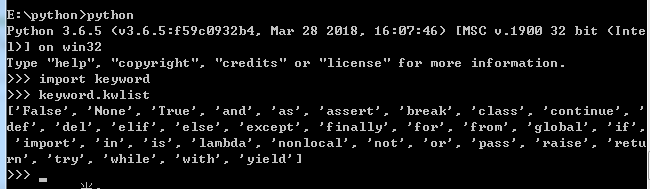#### 2. 打印

1). 写代码

# !/usr/bin/python3

# 第一个注释
print ("Hello, Python!")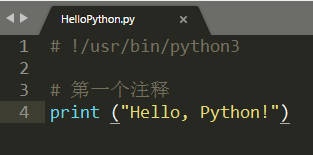2). 命令行运行

python helloPython.py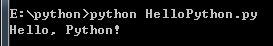#### 3. 注释

# !/usr/bin/python3

# 第一个注释
print ("Hello, Python!")

# 第一个注释
'''

'''
"""

"""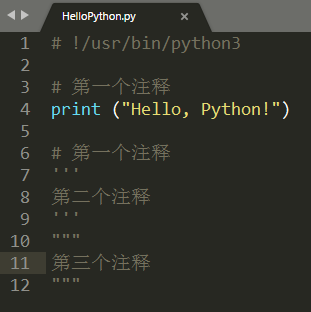#### 4. 缩进

python最具特色的就是使用缩进来表示代码块，不需要使用大括号 {} 。

if True:
print ("True")
else:
print ("False")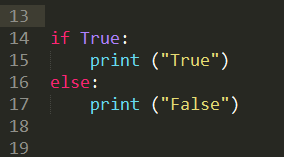#### 5. 多行语句

1). Python 通常是一行写完一条语句，但如果语句很长，我们可以使用反斜杠()来实现多行语句

total = item_one + \
item_two + \
item_three


2). 在 [], {}, 或 () 中的多行语句，不需要使用反斜杠()

total = ['item_one', 'item_two', 'item_three',
'item_four', 'item_five']


#### 6. 数据类型

1). 数字有四种类型：整数、布尔型、浮点数和复数

int(整数：1)
boolean(布尔类型：true)
float(浮点型：1.23)
complex(复数：1.1+2.2j)


2). 字符串

- python中单引号和双引号使用完全相同。
- 使用三引号('''或""")可以指定一个多行字符串。
- 转义符 '\'
- 反斜杠可以用来转义，使用r可以让反斜杠不发生转义。。 如 r"this is a line with \n" 则\n会显示，并不是换行。
- 按字面意义级联字符串，如"this " "is " "string"会被自动转换为this is string。
- 字符串可以用 + 运算符连接在一起，用 * 运算符重复。
- Python 中的字符串有两种索引方式，从左往右以 0 开始，从右往左以 -1 开始。
- Python中的字符串不能改变。
- Python 没有单独的字符类型，一个字符就是长度为 1 的字符串。
- 字符串的截取的语法格式如下：变量[头下标:尾下标]


str='Mazaiting'

# 输出字符串
print(str)
# 输出第一个到倒数第二个的所有字符
print(str[0:-1])
# 输出字符串第一个字符
print(str)
# 输出从第三个开始到第五个字符
print(str[2:5])
# 输出从第三个开始的所有字符
print(str[2:])
# 输出字符串两次
print(str * 2)
# 连接字符串
print(str + '你好')

print('-----------------------------')

# 使用反斜杠(\)+n转义特殊字符
print('hello\nworld')
# 使用字符串前面添加一个r，表示原始字符串，不会发生转义
print(r'hello\nworld')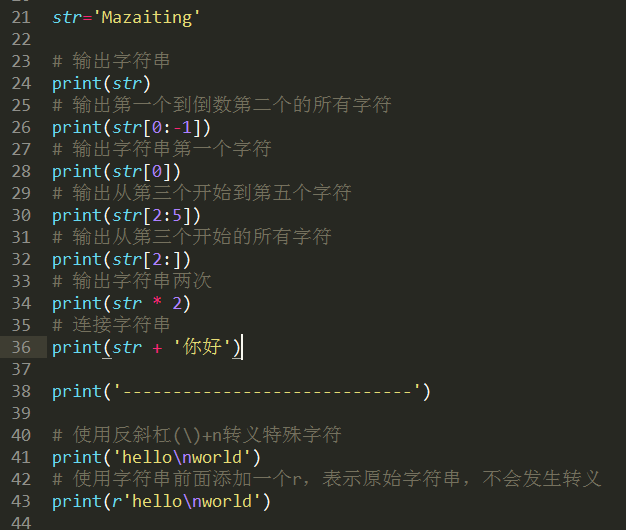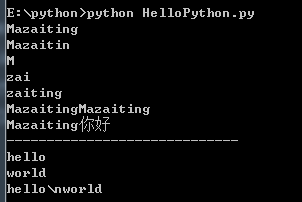#### 7. 等待用户输入

input("\n\n按下 enter 键后退出.")


#### 8. 同一行显示多条语句

import sys; x = 'mazaiting'; sys.stdout.write(x + '\n')


#### 9. 代码组

• 缩进相同的一组语句构成一个代码块，我们称之代码组。
• 像if、while、def和class这样的复合语句，首行以关键字开始，以冒号( : )结束，该行之后的一行或多行代码构成代码组。
• 我们将首行及后面的代码组称为一个子句(clause)。
if expression :
suite
elif expression :
suite
else :
suite


#### 10. 输出

x = "a"
y = "b"
# 换行输出
print(x)
print(y)
# 不换行输出
print(x, end = " ")
print(y, end = " ")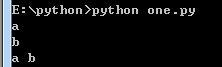#### 11. import 与 from...import

• 在 python 用 import 或者 from...import 来导入相应的模块。
• 将整个模块(somemodule)导入，格式为： import somemodule
• 从某个模块中导入某个函数,格式为： from somemodule import somefunction
• 从某个模块中导入多个函数,格式为： from somemodule import firstfunc, secondfunc, thirdfunc
• 将某个模块中的全部函数导入，格式为： from somemodule import *
示例1：
# 全部导入
import sys

print('=====================Python import mode====================')

print('命令行参数为：')

for i in sys.argv:
print(i)

print ('\n Python 路径为 ', sys.path)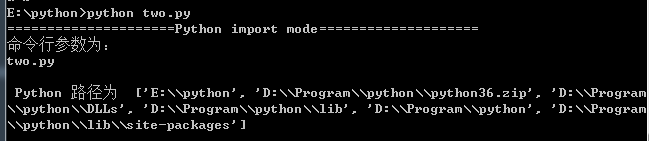# 导入sys模块的argv,path成员
from sys import argv,path
print('=====================Python import mode====================')

print ('path: ', path)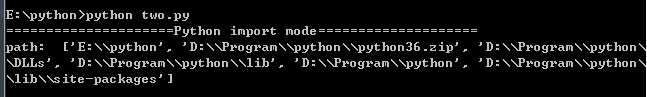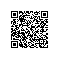使用钉钉扫一扫加入圈子
+ 订阅